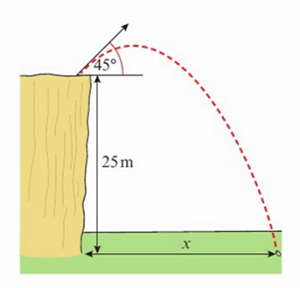Translate

An award winning sixth-form college in the heart of Central London offering a wide range of GCSE and A-Level courses

The quality of the curriculum, teaching and learners' achievements is excellent
ISI INSPECTION REPORT - APRIL 2023

T (+44) 20 7409 7273
Albemarle Independent College, 18 Dunraven Street, Mayfair, London W1K 7FE# Mathematics (PEARSON)

AS Course Outline

What is Mathematics AS?Mathematics is reasoning with quantities. If you are looking at studying a subject where data analysis, numerical aptitude or logical thought is valued, then an AS in Mathematics can provide an excellent introduction to these areas. AS Mathematics gives insight into the ways in which Mathematics can be used to model and analyse the world around us or create abstract ideas with far-reaching consequences. If you are thinking about continuing your study of Mathematics beyond AS level, then this course will give you an excellent insight into the mind-set and skills required. You will need to be a strong logical thinker with the ability to manipulate quantities both known and unknown. If you are able to achieve a 5 or above at GCSE then you stand a good chance of being a suitable candidate for AS Mathematics.

What Skills Will Students Develop When Studying AS Level Mathematics?

AS Level mathematics extends the approach of GCSE and challenges you to think on a more rigorous basis. You will be expected to solve problems with many stages, planning out and working your way to solutions neatly and concisely. A strong algebraic vein also runs through the course.

You will be introduced to all the fundamental functions of Mathematics in the AS course. Starting off with simple linear equations, the course builds up your knowledge so that you can analyse ‘general polynomials’ with higher powers of x, both graphically and algebraically. Trigonometry from GSCE will be built upon and a powerful algebraic technique called calculus is introduced.

You will study the Applied Mathematics: Mechanics and Statistics. In these you develop a sense of Mathematics as a tool for modelling the real world. You will discover the reasons for, as well as the restrictions of, mathematical models in a variety of contexts. In Mechanics, Newton's equations of force and motion, stability and vectors are the main fields of study. Statistics expands on the probability studied at GCSE and formalises the idea of a line of best fit. New concepts are introduced, such as the normal distribution, which is a distribution of results found throughout the natural world.

 MATHEMATICS (PEARSON) Summary of Content at AS Course Content Pure Mathematics: Algebra and functions; coordinate geometry, differentiation and integration, equation of a circle, trigonometry, exponentials and logarithms, vectors, geometric interpretation of calculus. Statistics: Statistical sampling, representing and summarising data, probability, correlation and regression, probability, statistical distributions and hypothesis testing. Mechanics: Modelling physical systems, quantities in mechanics, motion in a straight line, Newton's laws, applying vectors to 2D forces, moments.

Structure of the Course
Lessons are taught by specialist teachers who will guide you through worked examples and past papers questions. Students are required to take detailed notes to consolidate at home and use to complete homework assignments. Guidance will be given on exam technique. All components of the course are taught alongside one another.

 Core Textbooks Edexcel AS and A level Mathematics Pure Mathematics Year 1/AS Textbook Edexcel AS and A level Mathematics Statistics and Mechanics Year 1/AS Textbook Additional Reading Why do buses come in threes? Rice, Wyndham & Eastaway Zero: Biography of a dangerous idea Charles Seife 1089 and All That David Acheson